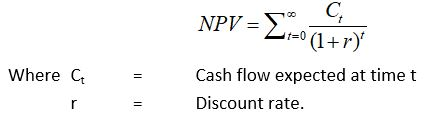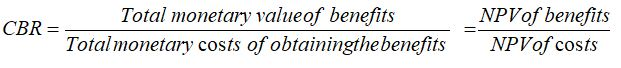## Value Impact Assessment

The Value Impact Assessment model considers operational costs (labour, maintenance, etc.), capital cost recovery, inflation, required return on investment and risk.

The model also calculates:
• Net Present Value (NPV)
• Sensitivity Analysis
• Cost to Benefit Ratio (CBR)

Net Present Value (NPV)
The NPV cost analysis method is mathematically expressed as:The model is able to calculate a Net Present Value (NPV) for the project lifespan. This parameter represents an absolute measure of viability associated with any given project. To infer financial feasibility, the NPV needs only to be greater than zero.

The NPV is normally calculated for multiple iterations, and includes standard deviations and a random number generator to the parameters to calculate the variance in the NPV. A more accurate reflection of the most likely NPV scenario can therefore be determined.

Sensitivity Analysis
A sensitivity analysis is also included to evaluate the risk of the proposed project. The sensitivity analysis tests the effect of each assumption on the NPV, by varying specific parameters (variables). The resulting change in the NPV per variable indicates the degree of sensitivity of the project to that variable. In general, the level of uncertainty associated with a parameter gives an indication of the risk of the project.Cost to Benefit Ratio (CBR)
Cost benefit analysis compares the economic benefits of a project to the economic costs of a project. Cost benefit analysis uses the Cost to Benefit Ratio or CBR to evaluate the attractiveness of a project. This ratio is defined as:In this instance, the CBR is calculated by dividing the NPV of the benefits by the NPV of the costs. For a favourable solution the ratio need only be greater than 1. Using the above model (i.e. inclusive of probability calculations using standard deviations, random numbers, etc.), multiple CBR iterations are performed.

Use of the aforementioned principles supports financially sound decision making for proposed projects.